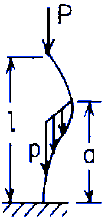Related Resources: calculators

Stepped straight bar under end load P and a distributed load of maximum value p at the bottom linearly decreasing to zero at a distance a from the bottom Upper end pinned, lower end fixed.
(a negative value for P/pa means the end load is tensile)NOTATION:

P = Load (force) (lbs, N);
(pa)' = Critical unit load (force per unit length), (lbs, N);
E = modulus of elasticity (force per unit area) (psi, Pa);
I = moment of inertia of cross section about central axis perpendicular to plane of buckling ( in4, mm4 )
l = Length of bar (in, mm),
K = Derived from ratios as follows (see table):
1.) a / l
2.) P / pa

Formulae:

(pa)' = K ( π2 E I ) / l2

(a negative value for P/pa means the end load is tensile)

References:

Roark's Formulas for Stress and Strain, Eight Edition